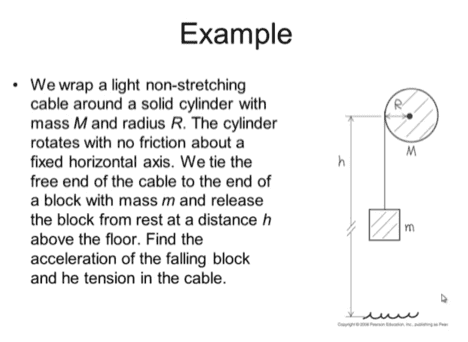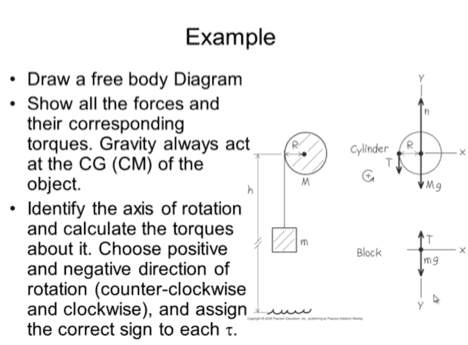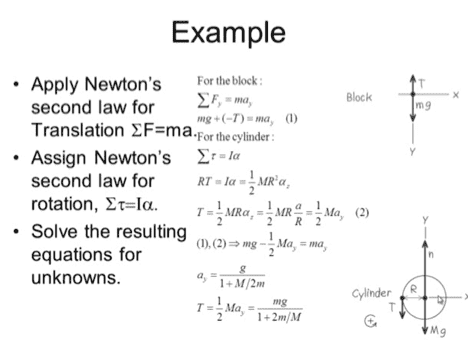# Angular Acceleration: Clarifying What αz is?

• oliampian
In summary, alpha z represents angular acceleration for rotation about the z-axis. The equation (2) states that alpha z = a/R, not alpha/R. The R cancels out and the "a" on the left is the same as ay. Dividing linear acceleration by the radius R results in angular acceleration.

## Homework StatementThis is not really a question on how to solve the problem, I'm just trying to get clarification on something. For angular acceleration, α, can someone explain to me what αz is? And why does αz = α / R = αy? I understand the rest of the problem, I just don't understand where or what αz is and how αz = α / R = αy. Thanks in advance for any help given!

oliampian said:
For angular acceleration, α, can someone explain to me what αz is?
##\alpha_z## apparently represents the angular acceleration for rotation about the z-axis.
And why does αz = α / R = αy?
The print in the picture is small and hard to read. In equation (2) I think it states that ## \alpha_z = \large \frac{a}{R}##, not ##\alpha_z = \large \frac{\alpha}{R}.##

TSny said:
##\alpha_z## apparently represents the angular acceleration for rotation about the z-axis.

The print in the picture is small and hard to read. In equation (2) I think it states that ## \alpha_z = \large \frac{a}{R}##, not ##\alpha_z = \large \frac{\alpha}{R}.##

Ohhh, you're right about the a/R not α/R. But can you explain to me why does a/R = ay?

oliampian said:
Ohhh, you're right about the a/R not α/R. But can you explain to me why does a/R = ay?
They aren't claiming that a/R = ay.

The R cancels: (1/2)MR(a/R) = (1/2)May. The "a" on the left is the same as ay.

TSny said:
They aren't claiming that a/R = ay.

The R cancels: (1/2)MR(a/R) = (1/2)May. The "a" on the left is the same as ay.

Ok I see. And just to clarify, if I divide a linear acceleration by the radius R then we get angular acceleration?

Nvm! Figured it out. Thank yoooou! :)

Last edited: